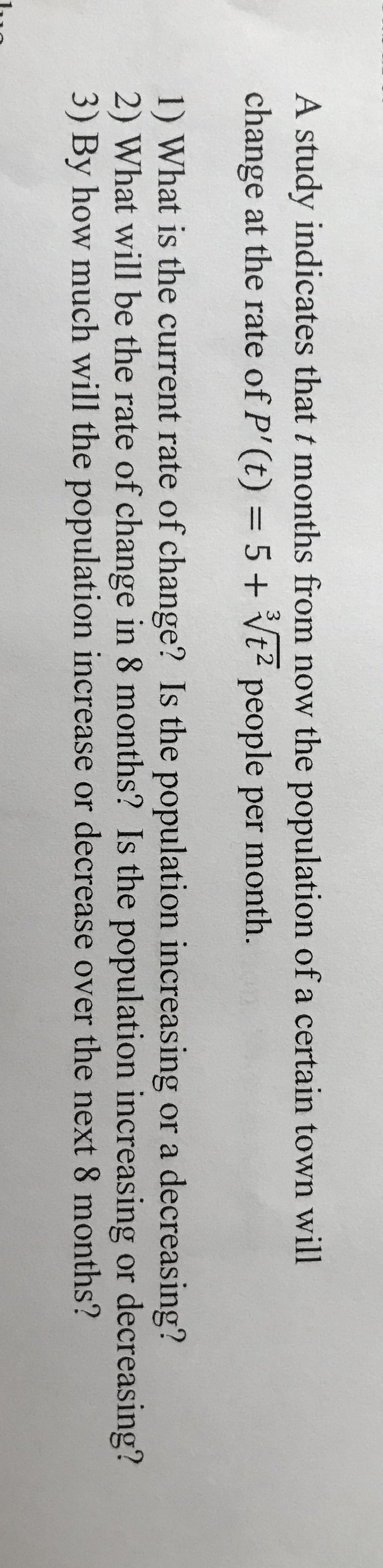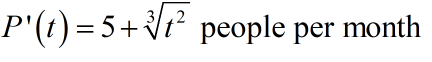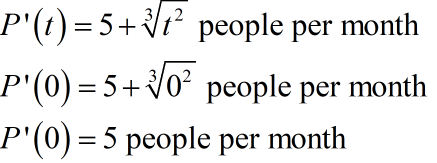# A study indicates that t months from now the population of a certain town willchange at the rate of P'(t) = 5+ Vt² people per month.31) What is the current rate of change? Is the population increasing or a decreasing?2) What will be the rate of change in 8 months? Is the population increasing or decreasing?3) By how much will the population increase or decrease over the next 8 months?

Question
2 viewshelp_outlineImage TranscriptioncloseA study indicates that t months from now the population of a certain town will change at the rate of P'(t) = 5+ Vt² people per month. 3 1) What is the current rate of change? Is the population increasing or a decreasing? 2) What will be the rate of change in 8 months? Is the population increasing or decreasing? 3) By how much will the population increase or decrease over the next 8 months? fullscreen
check_circle

Step 1

To determine the rate of change.

Step 2

Given:Step 3

(1)

For current rate of change comput P'(0) as,Since, P'(t) > 0 for t > 0 hence the population is increasing.

...

### Want to see the full answer?

See Solution

#### Want to see this answer and more?

Solutions are written by subject experts who are available 24/7. Questions are typically answered within 1 hour.*

See Solution
*Response times may vary by subject and question.
Tagged in